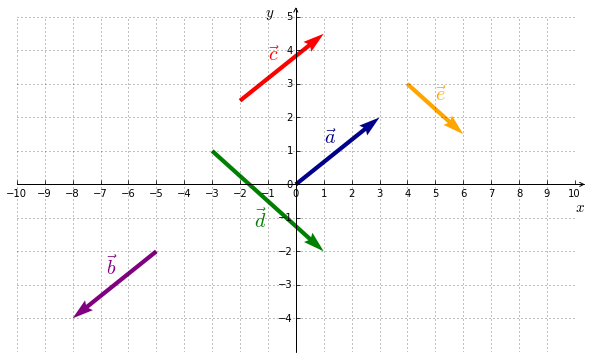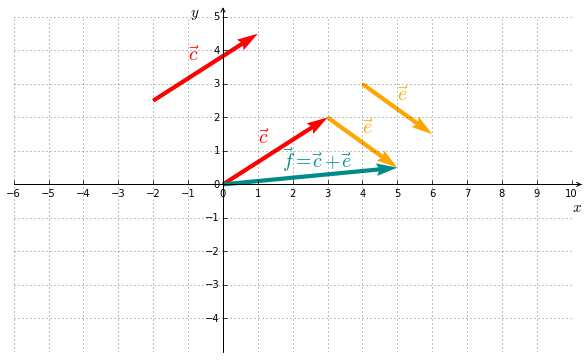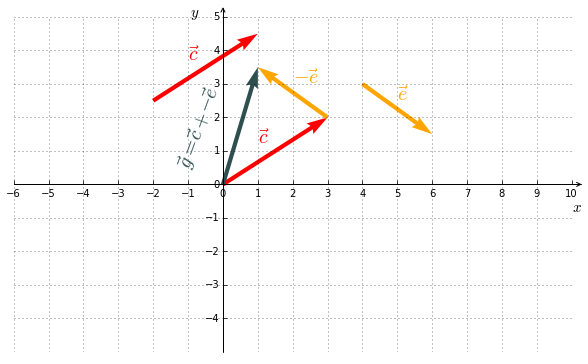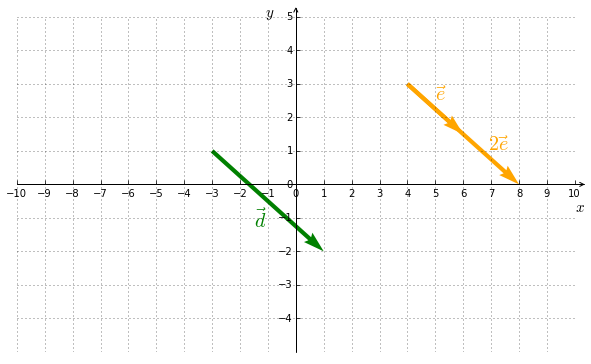## 1. Definition

Geometrically, a vector is an arrow having the three following characteristics :

• a direction (the line that contains it)
• a sense (top, right, bottom, left)
• a length (also called norm)

In Figure 5.1, we have the following :

• ${\color{navy}{\vec{a}}}$ et ${\color{red}{\vec{c}}}$ are equal. $\, {\color{navy}{\vec{a}}} ={\color{red}{\vec{c}}}$. The coordinates are : ${\color{red}{\vec{c}}}=\left( \begin{smallmatrix} 3 \\ 2 \end{smallmatrix} \right)$.
• ${\color{navy}{\vec{a}}}$ et ${\color{purple}{\vec{b}}}$ have the same direction, the same length but an opposite sense. $\, {\color{navy}{\vec{a}}} =-{\color{purple}{\vec{b}}}$.
• ${\color{green}{\vec{d}}}$ et ${\color{orange}{\vec{e}}}$ have the same direction, the same sense, but a different length. $\, {\color{green}{\vec{d}}} =2\,{\color{orange}{\vec{e}}}$.Figure 5.1 : vectors in $\mathbb{R}^2$

The addition of two vectors is defined as follows :

Geometrically, the sum of two vectors is obtained by putting them end to end, as showed in Figure 5.2. This is the so-called parallelogram law.

#### Example IFigure 5.2 : vector addition in $\mathbb{R}^2$

## 3. Subtraction

The vector subtraction is nothing but adding a vector whose sense is reversed.

#### Example IIFigure 5.3 : vector subtraction in $\mathbb{R}^2$

## 4. Multiplication by a scalar (number)

A vector multiplied by a scalar gives another vector. It is is defined as follows :

#### Example IIIFigure 5.4 : multiplication by a scalar in $\mathbb{R}^2$

## Recapitulation

Geometrically, a vector is an arrow having a direction, a sense and a length. Two vectors are equal if they have those same characteristics or, in other words, if they have the same coordinates.

Geometrically, a vector can been seen as a point. The concept of vector generalizes the concept of point.

If two vectors have the same direction, then they are multiples of one another, like $\vec{d}$ and $\vec{e}$ in Figure 5.4. In that case, they are said to be collinear or, more generally, linearly dependant.

More generally, a vector is an algebraic and abstract concept which is beyond the scope of the present course.